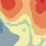# Semivariogram/covariance binned and average points

1867
2
06-07-2016 04:48 PMNew Contributor II

I am sorry if I am unaware of some very basic ideas of semivariogram. I am trying to understand the difference between binned points and averaged points. In this document (http://desktop.arcgis.com/en/arcmap/latest/extensions/geostatistical-analyst/fitting-a-model-to-the-...) it says: "Binned values are shown as red dots, and are generated by grouping (binning) empirical semivariogram/covariance points together using square cells that are one lag wide. Average points are shown as blue crosses, and are generated by binning empirical semivariogram/covariance points that fall within angular sectors."

My question is, what does it mean by "angular sectors".

Tags (1)
1 Solution

Accepted SolutionsbyEsri Regular Contributor

Both the red dots and the blue crosses are the result of binning empirical semivariances (or covariances), but the binning is done in different ways.  The red dots are binned from square cells, and the blue crosses are binned from angular sectors.

This paper details how this process is done.  To see pictures of the two binning methods, go to page 25.  Figure 2 shows the angular sectors (which create the blue crosses) and Figure 3 shows the square cells (which create the red dots).

2 RepliesbyEsri Regular Contributor

Both the red dots and the blue crosses are the result of binning empirical semivariances (or covariances), but the binning is done in different ways.  The red dots are binned from square cells, and the blue crosses are binned from angular sectors.

This paper details how this process is done.  To see pictures of the two binning methods, go to page 25.  Figure 2 shows the angular sectors (which create the blue crosses) and Figure 3 shows the square cells (which create the red dots).New Contributor II

Thank you so much. The paper exactly explains what I was trying to understand. Thanks.